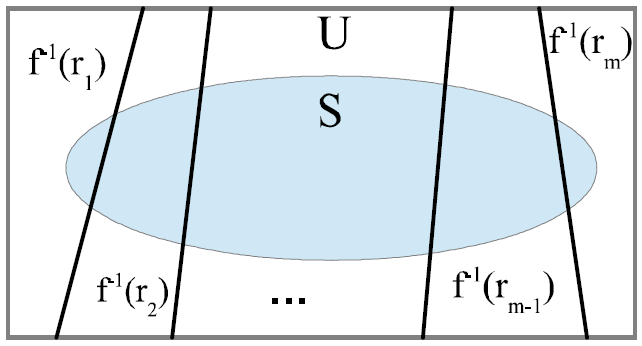# Book draft: Quantum Mechanics over SetsQuantum mechanics overs sets (QM/ℤ₂ or QM/Sets) is:
1) a pedagogical or `toy’ model of finite-dimensional quantum mechanics (QM/ℂ) that reproduces in the simplified setting of vector spaces over ℤ₂ the essentials of projective measurements, the double-slit experiment, the indeterminacy principle, entanglement, Bell’s Theorem, the statistics of indistinguishable particles, and so forth,
2) a non-commutative extension of finite probability theory that includes “superposition events” in addition to the usual classical events (subsets of the outcome set) and multiple equi-cardinal bases, and
3) a new objective indefiniteness approach to the old problem of interpreting quantum mechanics by highlighting the mathematics of indefiniteness vs. definiteness (i.e., partitions) and by showing what is important and what is not important (e.g., the wave interpretation) in the usual mathematics of QM/ℂ.